Home > Library > Statistics > Dependent and Independent Variables in Statistics

# Dependent and Independent Variables in Statistics

Published by at September 1st, 2021 , Revised On February 8, 2023

You might not assess an experiment or study accurately if you struggle with differentiating dependent and independent variables. Understanding these two terms is the key to successfully driving a research process as they determine the cause and effect in an experiment. And, that is why we are here to help you get through the stumbling block.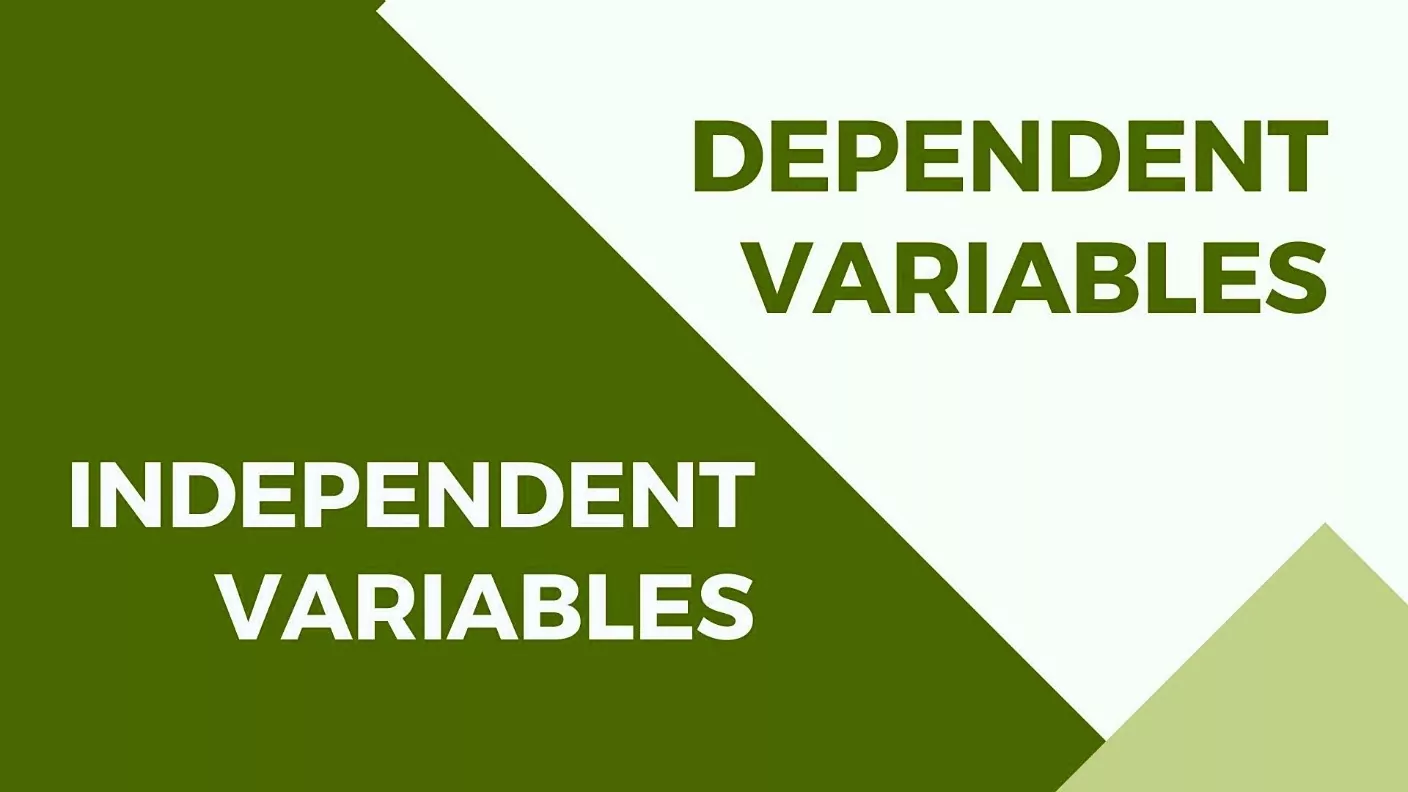Before we start with the key differences between dependent and independent variables, let us first look into what a variable is in the first place.

### What is a Variable in Statistics?

A variable in statistics is an unknown value that you are trying to measure. It can be anything from objects and things to feelings, time, events, or circumstances. For instance, if you are required to calculate the time for an object to move from point A to point B, variables here would be the time and speed of the object. Similarly, if you want to find how much people feel about different YouTube channels in today’s digitally advanced era, the variable, in this case, will be the YouTube channels people watch and their likes or dislikes (feelings).

Variables are divided into two main types that are imperative for every experiment: dependent variable and independent variable.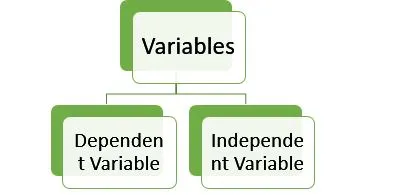### What is a Dependent Variable?

Can you guess from the name what a dependent variable is?

Well, that is correct!

A dependent variable is one that completely depends on another variable, mostly the independent one. This implies that the value of a dependent variable will change as soon as the value of the independent variable makes a shift.

The direction of this shift or change is determined by a function representing the relationship between independent and dependent variables.

Here is a mathematical term to exemplify this:

z = f (h) = 5x+2

In this equation, ‘z’ is the dependent variable, while ‘h’ is the independent variable. The ‘f(h)’ here is the function of the independent variable.

The dependent variable is sometimes called the predicted variable. We can conclude that the predictable variable measures the effect of the independent variable on the test units.

## What data collection best suits your research?

• Find out by hiring an expert from Research Prospect today!
• Despite how challenging the subject may be, we are here to help you.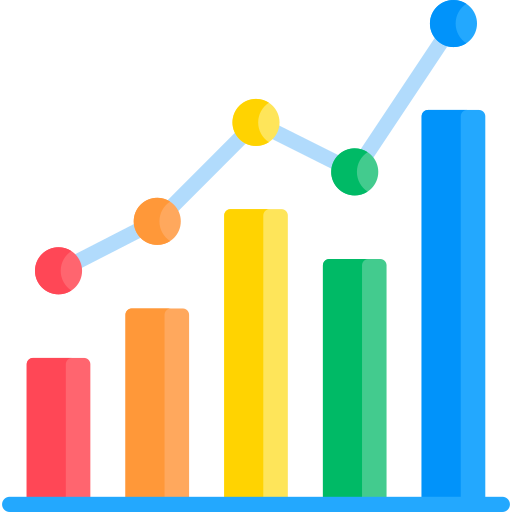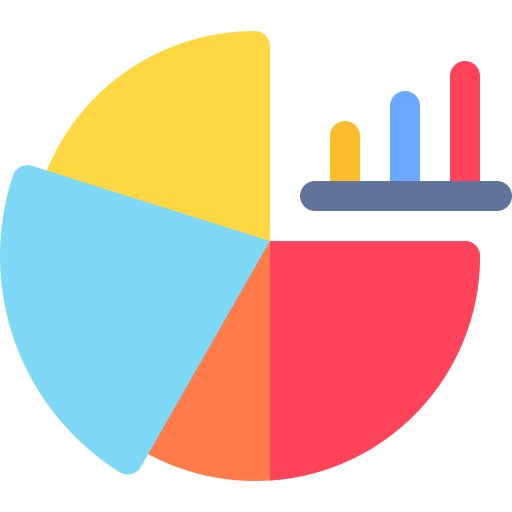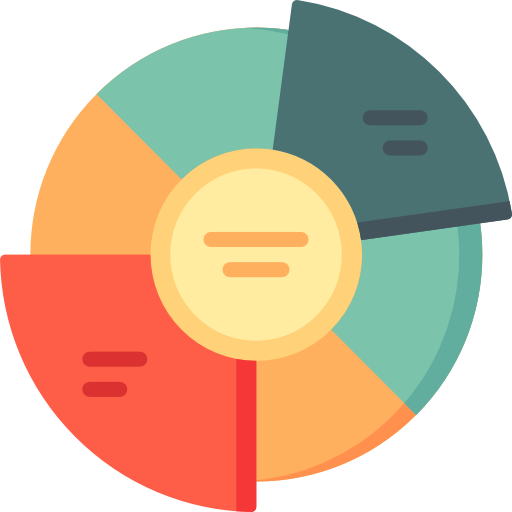### What is an Independent Variable?

An independent variable is one whose value does not change with the changing value of other variables. It is the variable that is not affected in the experiment. Researchers can either change the value themselves, or it changes on its own. But nothing else in the research or experiment can influence it.

An example of the independent variable is age and time. You can simply not make the time or age grow faster or slower, no matter what you do. These two are independent of everything else. Likewise, if we look at the example of an object moving from one point to another, explained earlier. We might notice that the time taken to get to a particular point will solely depend on how fast or slow it moves (speed), which is an independent variable. It is thus, called the predictor variable as well.

In other words, the independent variable in an experiment is what you change, while the dependent variable is what changes because of that.

## Dependent and Independent Variables in Experiments

We just discussed how the independent variable in an experiment is what the researcher can change while the dependent variable is the one changing because of the independent variable. Having that said, below are a few experiments to help you understand that.

Experiment 1:

Suppose you want to find out which fertilizer suits you best for your plants’ growth. You add a different brand of fertilizer to each of your plants and see how much time they take to grow.

Let’s see what the dependent and independent variables are in this experiment.

 Dependent Variable How tall the plant gets Independent Variable Brand of fertilizer

Experiment 2:

In this experiment, you want to see how the temperature of the sea impacts fish life. What you do is here take two aquariums, each containing water from a specific ocean site under a different temperature.

Let’s see what the dependent and independent variables are in this experiment.

 Dependent Variable Number of fish that survive Independent Variable Water temperature

You must be clear by now that the independent variables have zero effect on the temperature unless you decide to make certain amendments. But, if you change the values of the independent variables, in the above examples, the number of fish and the water temperature, the value of the dependent variables will change accordingly.

## Is the Statistics assignment pressure too much to handle?

### How about we handle it for you?

Put in the order right now in order to save yourself time, money, and nerves at the last minute.

## Dependent and Independent Variables in Graphs

Lastly, let us find out how to show these two variables on graphs.

Do graphs scare you? Because most people are seen avoiding them.

Don’t worry! They will not anymore. We have simplified graphs to the core for you.

Dependent and independent variables go in the same places when it comes to graphs. Always! The benefit to this is that you can instantly spot and evaluate which is the dependent variable and which is the independent one.

We will show these variables in the form of the x-axis, which is usually the horizontal axis, and the y-axis, usually being the vertical axis on the graph.

 X-axis Independent Variable Y-axis Dependent Variable

Example:

Let’s say you are to the number of extra hours at work against the increase in salary.

So, it will be “Extra Hours vs. Added Bonus/Money”.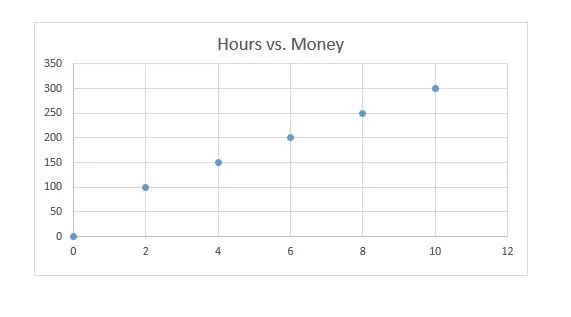This graph shows that if you increase the number of hours, the amount of extra money will also rise. The number of hours here is the independent variable because if it changes, the amount of bonus also changes.

Now there are many ways you can title a graph. However, the most common one is the “Independent vs. Dependent Variable.”

## FAQS About Independent and Dependent Variables

The independent variable is the one that does not have any effect on an experiment, while the dependent variable is one that can change with the changing value of the independent variable.

Variables are usually showed on the graph in the form of the x-axis and y-axis. The x-axis is the dependent variable, while the y-axis is the independent variable.

Suppose you want to find out which fertilizer suits best for your plants growth. You add a different brand of fertiliser to each of your plants and see how much time they take to grow.

• Dependent Variable: How tall the plant gets
• Independent Variable: Brand of fertilizerIngram is a dissertation specialist. He has a master's degree in data sciences. His research work aims to compare the various types of research methods used among academicians and researchers.